# An important calculation of the 7D number tau (circular version).

I really took the time to compose this post; basically it is not extremely difficult to understand. Everybody who once has done matrix diagonalization and is still familiar with the diverse concepts and ideas around that can understand what we are doing here.

It is the fact that it is seven dimensional that makes it hard to write down the calculations in a transparent manner. I think I have succeeded in that detail of transparency because at the end we have to multiply three of those large seven by seven matrices with each other and mostly that is asking for loosing oversight.

Luckily one of those matrices is a diagonal matrix and with a tiny trick we can avoid the bulk of the matrix calculations by calculating the conjugate of the number tau.

Just like in the complex plane where the conjugate of the number i equals -i, for tau goes the same.

Basically the numbers tau are always the logarithm of the first imaginary component. But check if the determinant is one because you can use the tau to craft an exponential curve that will go through all basis vectors with determinant one.

This post is 10 pictures long (size 550 x 775), in the beginning I use an applet for the numerical calculation of the matrix representation of the first imaginary unit in 7D space, here is the link:

Matrix logarithm calculator
http://calculator.vhex.net/calculator/linear-algebra/matrix-logarithm

Two years back in 2015 after I found the five dimensional numbers tau every now and then I typed in a higher dimensional imaginary unit and after that only staring at the screen of the computer: How to find those numbers as the log applet says…

The method as shown here can be applied in all dimensions and you now have a standard way of crafting exponential curves in all spaces you want. This method together with the modified Dirichlet kernels that provide always a parametrization of the exponential curve form a complete description.
Ok ok those modified Dirichlet kernels always have period pi while this way to calculation the log of the first imaginary unit is always related to the dimension (recall that the 7D first imaginary unit l has the property l^7 while for the complex multiplication in 7D space we have l^7 = -1), but it is very easy to fix the Dirichlet kernels to the proper period in the time domain you want.

The most difficult part of this post is in understanding the subtle choice for the eigenvalues of tau = log l, or better; choosing the eigenvalues of the matrix representation involved. That makes or breaks this method, if done wrong you end up with a giant pile of nonsense…

Have fun reading it and if this is your first time you encounter those matrices with all these roots of unity in them, take your time and once more: take your time.
If you have never seen a matrix like that it is very hard to understand this post in only one reading…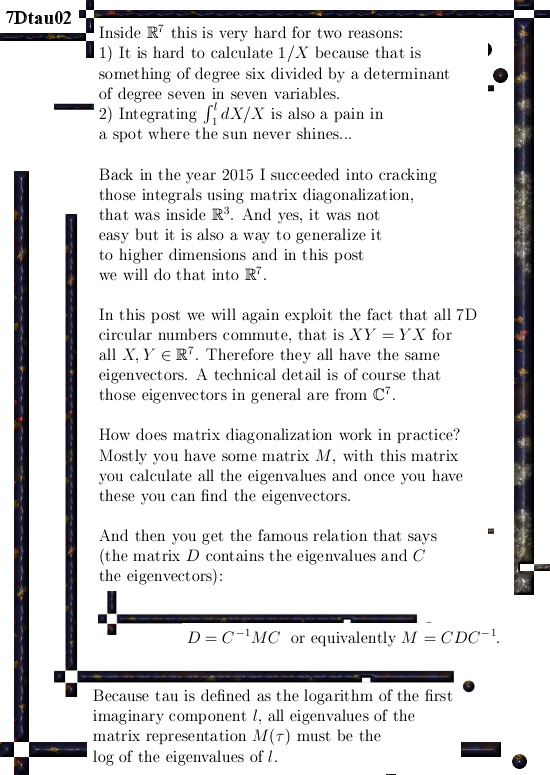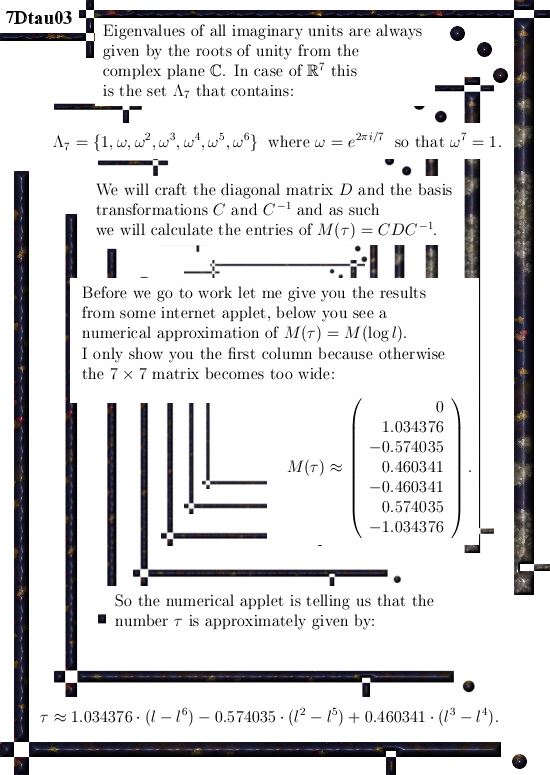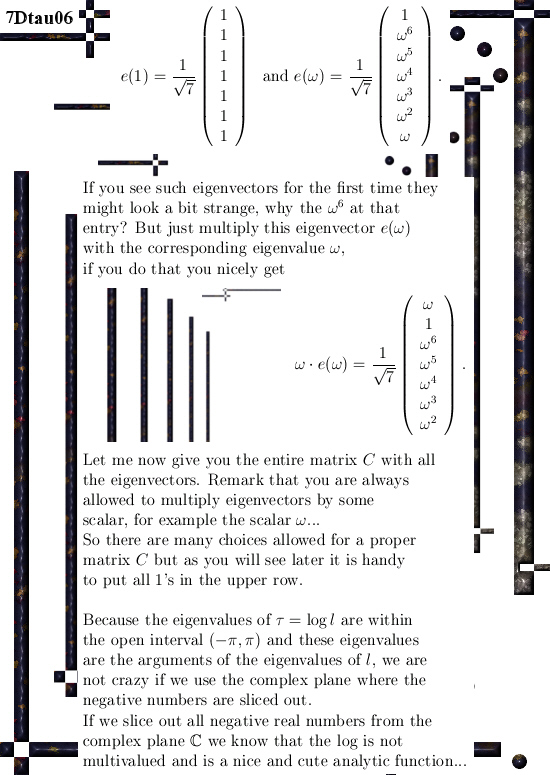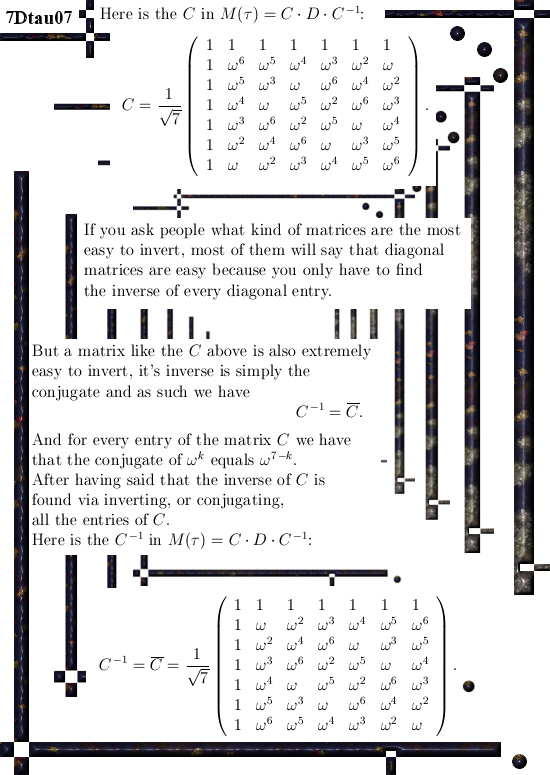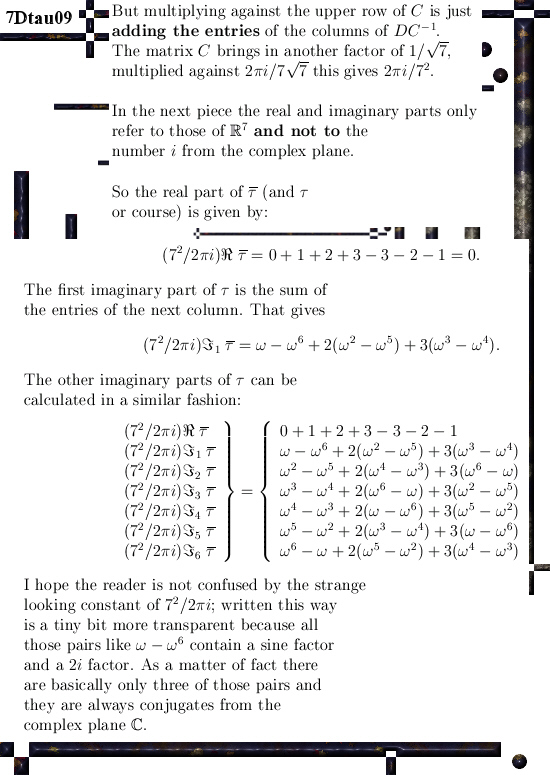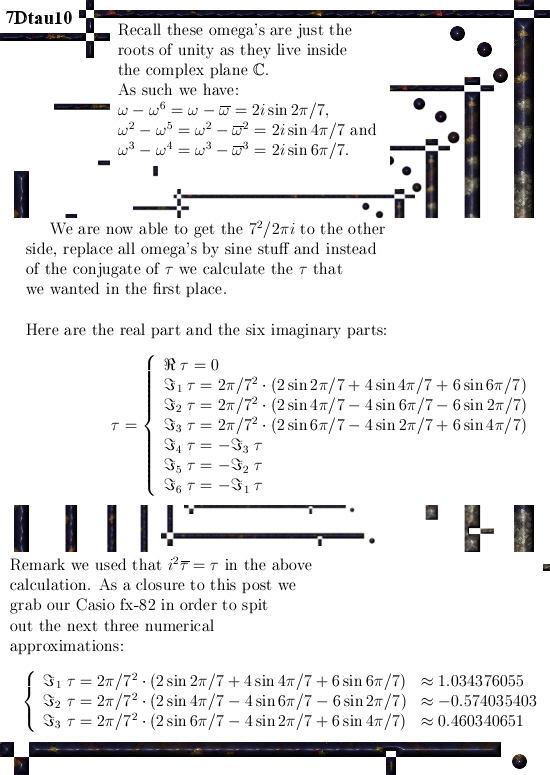I am glad all that staring to those numerical values is over and we have the onset of analytical understanding of how they are in terms of the angle 2 pi over 7.
The result is far from trivial; with the three or five dimensional case you can use other ways but the higher the dimension becomes the harder it gets.

This method that strongly relies on finding the correct diagonal matrix only becomes more difficult because the size of the matrices grows. So only the execution of the calculation becomes more cumbersome, the basic idea stays the same.

__________

I have no idea what the next post is going to be, may be a bit of magnetism because a few days back I got some good idea in explaining the behaviour of solar plasma included all those giant rings that shoot up and land in another spot of the sun.

And we also have those results from the Juno mission to Jupiter where the electrons also come from Jupiter itself without the guidance of electrical fields. But in the preprint archive I still cannot find only one work about it, that might be logical because often people do not write about stuff they don’t understand…

Ok, that was it. I hope you liked it & see ya around.